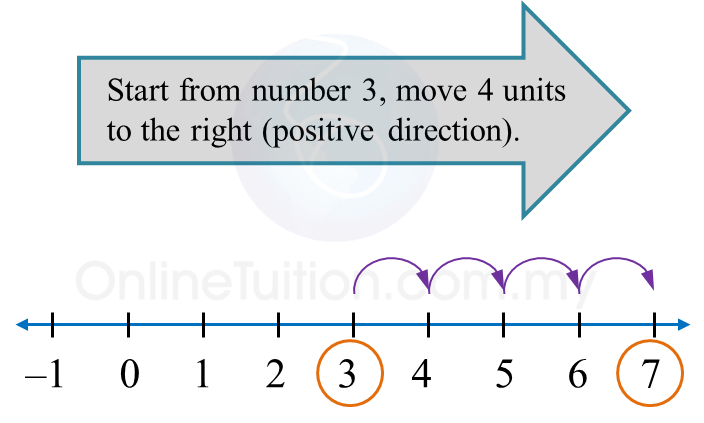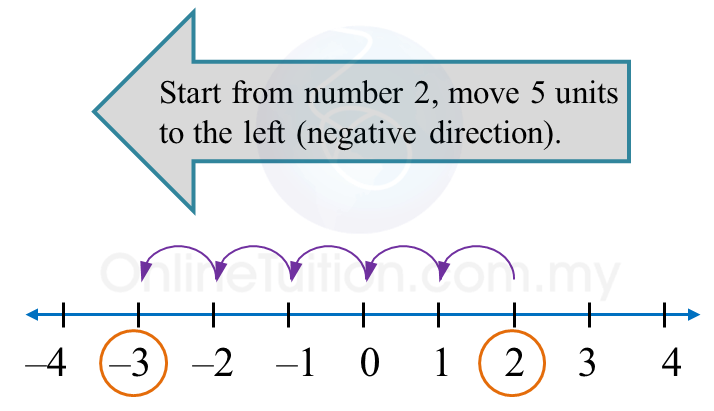6.2 Integers

6.2 Addition and Subtraction of Integers

6.2.1 Addition of Integers
1.
Addition of integers can be done on a number line.
(a) To add a positive integer:
Move to the right (positive direction) on the number line.

(b)
To add a negative integer:
Move to the left (negative direction) on the number line.2. Integers
with the same signs are called integers with like signs.
Example:
2 and 7, –25 and –5.

3. Integers with the different signs are called integers with unlike signs.
Example:
+2 and –7, –25 and 5.

Example 1:

Solve the following.
(a) 3 + (+4)
(b) 2 + (–5)

Solution:
(a)Therefore,
3 + (+4) = 3 + 4
= 7

(b)Therefore,
2 + (–5)
= –3

6.2.3 Multiplication and Division of Integers
1. Multiplication and division of like signs gives (+)

$\overline{)\begin{array}{l}\text{}\left(+\right)×\left(+\right)=+\text{}\left(+\right)÷\left(+\right)=+\text{}\\ \text{}\left(-\right)×\left(-\right)=+\text{}\left(-\right)÷\left(-\right)=+\end{array}}$

2.
Multiplication and division of unlike signs gives ()

$\overline{)\begin{array}{l}\text{}\left(+\right)×\left(-\right)=-\text{}\left(+\right)÷\left(-\right)=-\text{}\\ \text{}\left(-\right)×\left(+\right)=-\text{}\left(-\right)÷\left(+\right)=-\end{array}}$

Example:
(a) –25 ÷ 5 = –5
(b)   8 × (–5) = –40